# Introduction

Monocular surface reconstruction of deformable objects is a challenging problem which has known renewed interest during the past few years. This problem is fundamentally ill-posed because of the depth ambiguities; there are virtually an infinite number of 3D surfaces that have exactly the same projection. It is thus necessary to use additional constraints ensuring the consistency of the reconstructed surface.

In this chapter, we present two algorithms for monocular reconstruction of deformable and inextensible surfaces under some general assumptions. First, we consider the template-based case. Reconstruction is achieved from point correspondences between an input image and a template image showing a flat reference shape from a fronto-parallel point of view. Second, we suppose the intrinsic parameters of the camera to be known. Third, we assume that the camera is a perspective camera. These are common assumptions (144,166,176).

Over the years, different types of constraints have been proposed to disambiguate the problem of monocular reconstruction of deformable surfaces. They can be divided into two main categories: the statistical and the physical constraints. For instance, the methods relying on the low-rank factorization paradigm (195,17,,28,29,58,138) can be classified as statistical approaches. Learning approaches such as (75,166,163,165) also belong to the statistical approaches. Work such as (166), where the reconstructed surface is represented as a linear combination of inextensible deformation modes, is also a statistical approach. Physical constraints include spatial and temporal priors on the surface to reconstruct (88,153). Statistical and physical priors can be combined (17,58). A physical prior of particular interest is the hypothesis of having an inextensible surface (164,144,176,166). In this chapter, we consider this type of surface. This hypothesis means that the geodesics on the surface may not change their length across time. However, computing geodesics is generally hard to achieve and it is even more difficult to incorporate such constraints in a reconstruction algorithm. There exist several approaches to approximate this type of constraint. For instance, if the points are sufficiently close together, the geodesic between two 3D points on the surface can be approximated by the Euclidean distance (175). An efficient approximation consists in saying that the geodesic distance between two points is an upper bound to the Euclidean distance (164,144).

Algorithms for monocular reconstruction of deformable surfaces can also be categorized according to the type of surface model (or representation) they use. The point-wise methods utilize a sparse representation of the 3D surface, i.e. they only retrieve the 3D positions of the data points (144). Other methods use more complex surface models such as triangular meshes (164,166) or smooth surfaces such as Thin-Plate Splines (11,144). In this latter case, the 3D surface is represented as a parametric 2D-3D map between the template image space and the 3D space. Smooth surfaces are generally obtained by fitting a parametric model to a sparse set of reconstructed 3D points: the smooth surface is not actually used in the 3D reconstruction process. In this chapter, we propose an algorithm that directly estimate a smooth 3D surface based on Free-Form Deformations (162). Having an inextensible surface means that the surface must be everywhere a local isometry. This induces conditions on the Jacobian matrix of the 2D-3D map. We show that these conditions can be integrated in a non-linear least-squares minimization problem along with some other constraints that force the consistency between the reconstructed surface and the point correspondences. Such a problem can be solved using an iterative optimization procedure such as Levenberg-Marquardt that we initialize using a point-wise reconstruction algorithm. Our approach is highly effective in the sense that it outperforms previous approaches in term of accuracy of the reconstructed surface and in terms of inextensibility.

Another important aspect in monocular reconstruction of deformable surfaces is the way noise is handled. It can be accounted for in the template image (144) or in the input image (166). There exist different approaches for handling the noise. For instance, one can minimize a reprojection error, i.e. the distance between the data points of the input image and the projection of the reconstructed 3D points. It is also possible to hypothesize maximal inaccuracies in the data points. We propose a point-wise approach that accounts for noise in both the template and the input images. This approach is formulated as a second-order cone program (SOCP) (25).

Table 7.1: Summary of the notation used in this chapter.
 Notation Description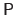Matrix of the intrinsic parameters of the camera () (The camera is assumed to be at the coordinate origin, so the matrixmay be assumed to be square and invertible.)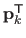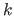th row of the matrix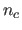Number of point correspondencesth point in the template image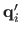th point in the input image;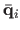Pointin homogeneous coordinatesSightline corresponding to the point(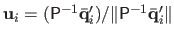)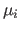Depth of the point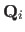Reconstructed 3D point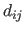Euclidean distance between pointsand()True value of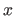(for)

Contributions to Parametric Image Registration and 3D Surface Reconstruction (Ph.D. dissertation, November 2010) - Florent Brunet
Webpage generated on July 2011